Next: Problem 1 Up: Local Star Formation Process Previous: Local Star Formation Process   Contents

# Hydrostatic Balance

Consider a hydrostatic balance of isothermal cloud. By the gas density,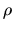, the isothermal sound speed,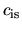, and the gravitational potential,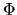, the force balance is written as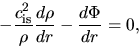(4.1)

and the gravity is calculated from a density distribution as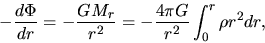(4.2)

for a spherical symmetric cloud, where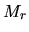represents the mass contained inside the radius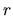. The expression for a cylindrical cloud is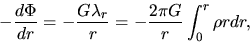(4.3)

where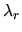represents the mass per unit length within a cylinder of radius being.

For the spherical symmetric case, the equation becomes the Lane-Emden equation with the polytropic index of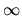(see Appendix C.1). This has no analytic solutions. However, the numerical integration gives us a solution shown in Figure 4.1 (left). Only in a limiting case with the infinite central density, the solution is expressed as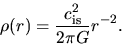(4.4)

Increasing the central density, the solution reaches the above Singular Isothermal Sphere (SIS) solution.

On the other hand, a cylindrical cloud has an analytic solution (Ostriker 1964) as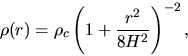(4.5)

where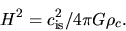(4.6)

Far from the cloud symmetric axis, the distribution of equation (4.5) gives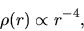(4.7)

while the spherical symmetric cloud has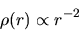(4.8)

distribution.

SubsectionsNext: Problem 1 Up: Local Star Formation Process Previous: Local Star Formation Process   Contents
Kohji Tomisaka 2007-07-08